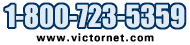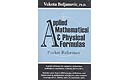Metalworking Tools and Supplies

Model # Qty

# Applied Mathematical and Physical Formulas

## Applied Mathematical and Physical Formulas Pocket ReferenceClick for a Bigger Picture
Applied Mathematical and Physical Formulas Pocket Reference
Vukota Boljanovic
361 pages, Illustrated, 5 x 7
Published: August 2006

OVERVIEW
If formulas have always been your nemeses, this book is for you! Thoroughly practical and authoritative, this book brings together, in three parts, more than 1,300 formulas, rules, and figures to simplify, review, or to refresh the users memory of what he/she studied in school. This pocket reference shows how to solve every kind of math and physics problem youre likely to encounter in school and on-the-job, and it explains simply and easily how to find answers fast, learn key formulas and definitions, study quickly and learn more effectively--from fundamental mathematical rules to physical definitions and constants.

FEATURES
* Presents all formulas, rules, and definitions precisely, simply, and clearly.
* Covers metric units of measurement, U.S. units of measurement (USCS), tables of equivalents metrics and USCS units.
* Presents all formulas, rules, and definitions precisely, simply, and clearly.
* Covers metric units of measurement, U.S. units of measurement (USCS), tables of equivalents metrics and USCS units.
* Reviews the fundamentals of algebra, geometry, trigonometry, and analytical geometry.
* Presents the application of differential equations and integral calculus.
* Solves problems concerning simple interest, compound interest, effective rate, annuity, amortization of loans , and sinking fund payment.
* Shows the comparative advantages of binomial distribution, standard distribution, Poisson distribution, and normal distribution.
* Includes most used definitions and formulas of kinematics, dynamics, statics, mechanics of fluids, thermal variable of state, thermodynamics, electricity and magnetism, light, and basic definition of atomic and nuclear physics.
* Offers most used fundamentals of physical constants.
* Reviews the fundamentals of algebra, geometry, trigonometry, and analytical geometry.
* Presents the application of differential equations and integral calculus.
* Solves problems concerning simple interest, compound interest, effective rate, annuity, amortization of loans , and sinking fund payment.
* Shows the comparative advantages of binomial distribution, standard distribution, Poisson distribution, and normal distribution.
* Includes most used definitions and formulas of kinematics, dynamics, statics, mechanics of fluids, thermal variable of state, thermodynamics, electricity and magnetism, light, and basic definition of atomic and nuclear physics.
* Offers most used fundamentals of physical constants.

CONTENTS
Part I: Units
* International System of Units
* Metric Units of Measurement
* U.S. Units of Measurements
* Tables of Equivalents

Part II: Mathematics
* Algebra
* Geometry
* Trigonometry
* Analytical
* Geometry
* Mathematics of Finance
* Calculus Statistics

Part III: Physics
* Mechanics
* Mechanics of Fluid
* Temperature and Heat
* Electricity and Magnetism
* Light
* Wave Motion and Sound

Appendix
Index
Quantity Price Model Description

\$35.90 BK-AM
Applied Math and Physical Formulas